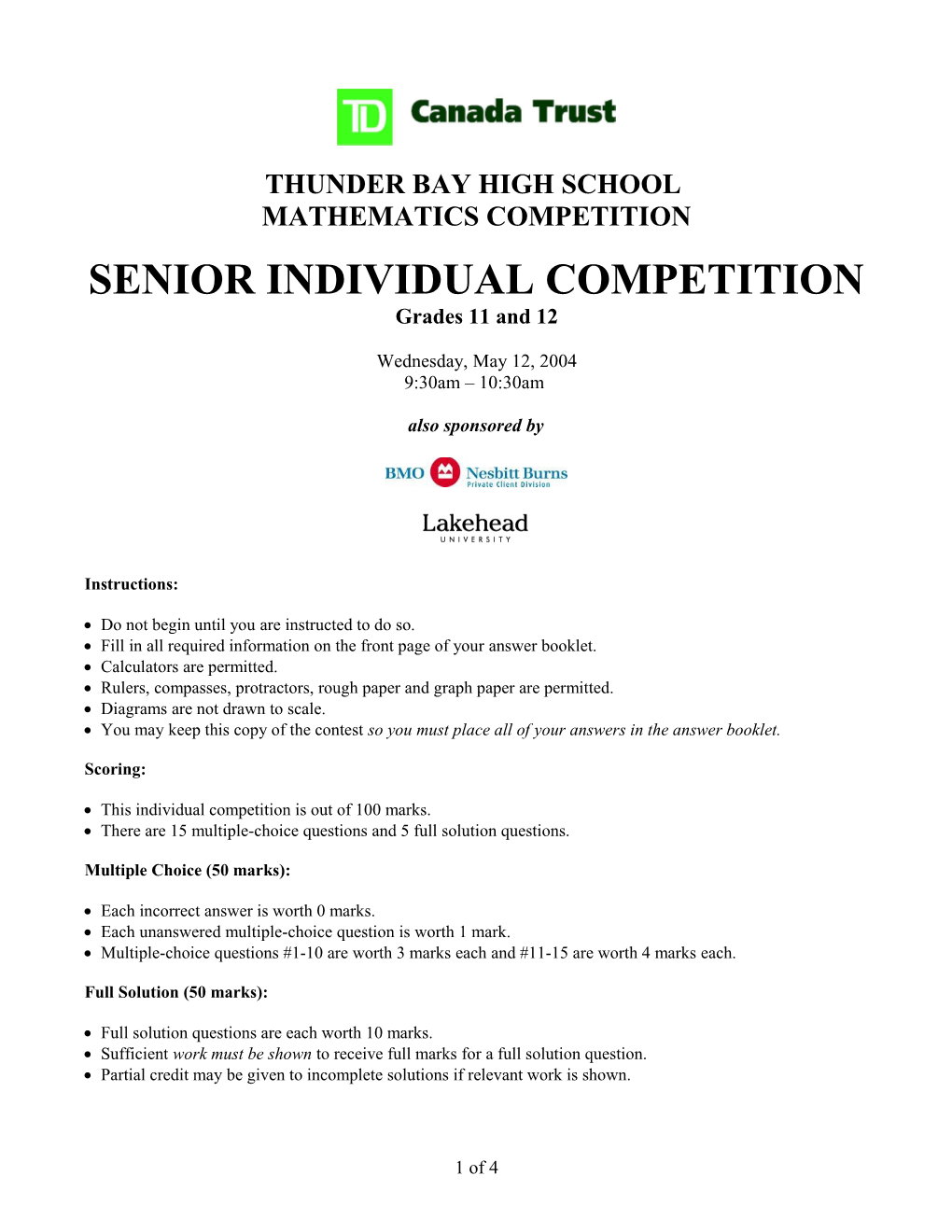# Thunder Bay High School## MATHEMATICS COMPETITION

### SENIOR INDIVIDUAL COMPETITION

Wednesday, May 12, 2004

9:30am – 10:30am

Instructions:

 Do not begin until you are instructed to do so.

 Fill in all required information on the front page of your answer booklet.

 Calculators are permitted.

 Rulers, compasses, protractors, rough paper and graph paper are permitted.

 Diagrams are not drawn to scale.

 You may keep this copy of the contest so you must place all of your answers in the answer booklet.

Scoring:

 This individual competition is out of 100 marks.

 There are 15 multiple-choice questions and 5 full solution questions.

Multiple Choice (50 marks):

 Each incorrect answer is worth 0 marks.

 Each unanswered multiple-choice question is worth 1 mark.

 Multiple-choice questions #1-10 are worth 3 marks each and #11-15 are worth 4 marks each.

Full Solution (50 marks):

 Full solution questions are each worth 10 marks.

 Sufficient work must be shown to receive full marks for a full solution question.

 Partial credit may be given to incomplete solutions if relevant work is shown.

Multiple Choice (50 Marks)

Place all answers in the multiple-choice boxes on the front page of the answer booklet.

______

Questions #1-10 below are worth:3 marks for a correct answer

1 mark for a blank answer

0 marks for an incorrect answer

1.The value of when x = 1 is

(A) (B)(C) 1(D) 2.5(E) 3.14

2.Yesterday, the reading on Howard’s electricity meter was 098657 units. He was shocked to realize that all six of the digits are different. How many more units of electricity will he use before the next time all the digits are different?

(A) 1(B) 4(C) 14(D) 55(E) 3688

3.Kathleen has two strange dice. The faces of each die show the numbers 1 to 6 as usual, but the odd numbers are negative (ie. –1, -3, -5 instead of 1, 3, 5). If she throws the two dice, which total cannot be achieved?

(A) 3(B) 4(C) 5(D) 7(E) 8

4.Let S(n) be the sum of the digits of the positive integer n. What is S(S(68795))?

(A) 5(B) 8(C) 20(D) 35(E) 114

5.The sum of the digits of the two-digit perfect square whose digits have a product that is also a perfect square is

(A) 11(B) 12(C) 13(D) 14(E) 15

6. The value of is

(A) 3/4(B) 4/5(C) 5/6(D) 6/7(E) 6/5

7.Suppose that your height this year is 10% more than it was last year, and last year your height was 20% more than it was the year before. By what percentage has your height increased during the last two years?

(A) 30%(B) 31%(C) 32%(D) 33%(E) 35%

8. Let a binary operation * on ordered pairs of integers be defined by (a,b)*(c,d) = (a-c,b+d). Then, if (3,2)*(0,0) and (x,y)*(3,2) represent identical pairs, x equals

(A) –3(B) 0(C) 2(D) 3(E) 6

9.The smallest positive integer x for which , where is an integer, is

(A) 1050(B) 1260(C) (D) 7350(E) 44100

10. As it is usually defined, . The largest prime number that divides is

(A) 19(B) 7(C) 20! + 1(D) (E) 167

______

Questions #11-15 below are worth:4 marks for a correct answer

1 mark for a blank answer

0 marks for an incorrect answer

11. The number is made out of 2004 digits all equal to 1. What is the sum of the digits of the number ?

(A) 10000(B) 10015(C) 12024(D) 14026(E)

12. A rectangle contains three circles as in the diagram below, all tangent to the rectangle and to each other. If the radius of the larger circle is 2, what is the width of the rectangle?

(A) (B) (C) (D) 6(E)

13.The increasing sequence 2,3,5,6,7,10,11…consists of all positive integers that are neither the square nor the cube of a positive integer. The 500th term of this sequence is

(A) 526(B) 527(C) 528(D) 529(E) 530

14.Let and be arithmetic series such that = 25, = 75 and . What is the sum of the first one hundred terms of the series?

(A) 0(B) 100(C) 10000(D) 505000(E) 2004

15.Let . Then the number of positive integer divisors of is

(A) 3(B) 5(C) 69(D) 125(E) 216

______

Full Solution (50 Marks)

Each question is worth 10 marks.

You must show sufficient work to receive full marks, but if you do not completely answer a question you may still receive partial marks for showing work. So show your work!

______

1.A circle and a parabola are drawn on a piece of paper. What is the largest number of regions into which they divide the paper? Why can’t they divide the paper into more regions than this?

2.Find the coefficient of in the polynomial expansion of .

3.Of 28 students taking at least one subject, the number taking Mathematics and English only equals the number taking Mathematics only. No student takes English or History only, and six students take Mathematics and History, but no English. The number taking English and History only is five times the number taking all three subjects. If the number taking all three subjects is even and non-zero, what is the number of students taking Mathematics and English only?

4.What is the value of ?

5.Solve the equation .

1 of 4